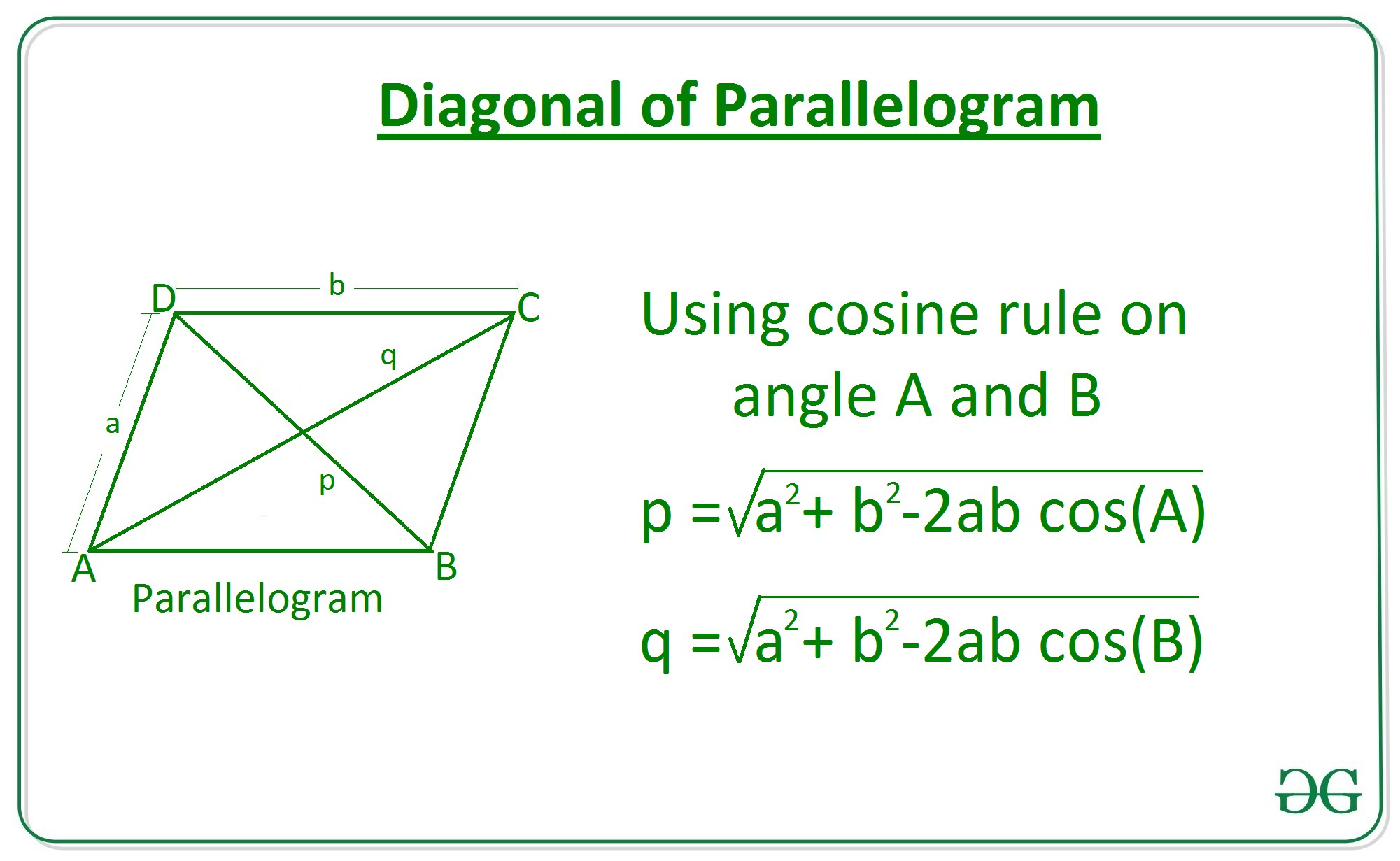# Length of diagonal of a parallelogram using adjacent sides and angle between them

Given two integers a and b where a and b represents the length of adjacent sides of a parallelogram and an angle 0 between them, the task is to find the length of diagonal of the parallelogram.

Examples:

Input: a = 6, b = 10, 0=30
Output: 6.14

Input: a = 3, b = 5, 0=45
Output: 3.58

Approach: Consider a parallelogram ABCD with sides a and b, now apply cosine rule at angle A in the triangle ABD to find the length of diagonal p, similarly find diagonal q from triangle ABC.Therefore the diagonals is given by:## C++

 // C++ program to find length  // Of diagonal of a parallelogram  // Using sides and angle between them.  #include  using namespace std;  #define PI 3.147     // Function to return the length  // Of diagonal of a parallelogram  // using sides and angle between them.  double Length_Diagonal(int a, int b, double theta)  {      double diagonal = sqrt((pow(a, 2) + pow(b, 2)) -                         2 * a * b * cos(theta * (PI / 180)));         return diagonal;  }     // Driver Code  int main()  {         // Given sides      int a = 3;      int b = 5;         // Given angle      double theta = 45;         // Function call      double ans = Length_Diagonal(a, b, theta);         // Print the final answer      printf("%.2f", ans);  }     // This code is contributed by Amit Katiyar

## Java

 // Java program to find length   // Of diagonal of a parallelogram   // Using sides and angle between them.  class GFG{     // Function to return the length  // Of diagonal of a parallelogram  // using sides and angle between them.  static double Length_Diagonal(int a, int b,                                double theta)  {      double diagonal = Math.sqrt((Math.pow(a, 2) +                                    Math.pow(b, 2)) -                                    2 * a * b *                                    Math.cos(theta *                                    (Math.PI / 180)));         return diagonal;  }     // Driver Code  public static void main(String[] args)  {             // Given sides      int a = 3;      int b = 5;         // Given angle      double theta = 45;         // Function call      double ans = Length_Diagonal(a, b, theta);         // Print the final answer      System.out.printf("%.2f", ans);  }  }     // This code is contributed by amal kumar choubey

## Python3

 # Python3 Program to find length   # Of diagonal of a parallelogram   # Using sides and angle between them.     import math         # Function to return the length  # Of diagonal of a parallelogram   # using sides and angle between them.    def Length_Diagonal(a, b, theta):             diagonal = math.sqrt( ((a**2) + (b**2))      - 2 * a*b * math.cos(math.radians(theta)))             return diagonal         # Driver Code     # Given Sides  a = 3 b = 5    # Given Angle  theta = 45      # Function Call    ans = Length_Diagonal(a, b, theta)         # Print the final answer  print(round(ans, 2))

## C#

 // C# program to find length   // Of diagonal of a parallelogram   // Using sides and angle between them.  using System;     class GFG{     // Function to return the length  // Of diagonal of a parallelogram  // using sides and angle between them.  static double Length_Diagonal(int a, int b,                                double theta)  {      double diagonal = Math.Sqrt((Math.Pow(a, 2) +                                    Math.Pow(b, 2)) -                                    2 * a * b *                                    Math.Cos(theta *                                   (Math.PI / 180)));         return diagonal;  }     // Driver Code  public static void Main(String[] args)  {             // Given sides      int a = 3;      int b = 5;         // Given angle      double theta = 45;         // Function call      double ans = Length_Diagonal(a, b, theta);         // Print the readonly answer      Console.Write("{0:F2}", ans);  }  }     // This code is contributed by amal kumar choubey

Output:

3.58


Time Complexity: O(1)
Auxiliary Space: O(1)

Attention reader! Don’t stop learning now. Get hold of all the important DSA concepts with the DSA Self Paced Course at a student-friendly price and become industry ready.

My Personal Notes arrow_drop_upCheck out this Author's contributed articles.

If you like GeeksforGeeks and would like to contribute, you can also write an article using contribute.geeksforgeeks.org or mail your article to contribute@geeksforgeeks.org. See your article appearing on the GeeksforGeeks main page and help other Geeks.

Please Improve this article if you find anything incorrect by clicking on the "Improve Article" button below.

Article Tags :
Practice Tags :

1

Please write to us at contribute@geeksforgeeks.org to report any issue with the above content.